# Optimization Efficiency of the Aircraft Wing of Cessna 172 Skyhawk by Absorbent Adverse Pressure Using Tangential Suction Slot Without Vacuum Device

Optimization Efficiency of the Aircraft Wing of Cessna 172 Skyhawk by Absorbent Adverse Pressure Using Tangential Suction Slot Without Vacuum Device

Naseer A. Mousa Osam H. Attia Hussein A. MahmoodNor Mariah Adam

Department of Reconstruction and Projects - University of Baghdad, Baghdad 10071, Iraq

Department of Chemical Engineering-Faculty of Engineering-UPM 43400, Malaysia

Corresponding Author Email:
Page:
731-738
|
DOI:
https://doi.org/10.18280/mmep.090320
31 March 2022
|
Accepted:
7 June 2022
|
Published:
30 June 2022
| Citation

OPEN ACCESS

Abstract:

Efficiency of a wing is sufficient at stall angle of attack and breakdown by effecting turbulent flow intensity on upper wing surface which is destroyed the airflow pattern and generated adverse pressure, backflow airstream and forward transition separation point movement closely to the leading edge of the wing. To enhancement a wing efficiency factor at high angle of attacks in this research is using partially sucking adverse pressure to delay the separation point to control the backflow rate and expelling it through the wing tips ends using tangential suction slot channel without using any mechanical means depending on differential static pressure between adverse pressure and the static pressure generated on both win tips ends. Numerical CFD analysis has been managed the case study of the effect suction slot channel located on the upper wing surface at (70%) of the wing chord from the leading edge as a proposed modified wing model of the Cessna 172 Skyhawk half wing at angle of attacks (10°, 12°, 14°, 16°, 18°, 20°, 22°, 24°). The analysis study was conducted at stall speed (90 km/hr.) without flaps where it is equal (25 m/sec). The results of the analytical shows at the range of angle of attacks were chosen between (10° to 24°), lift coefficient CL increased (3.757%), drag coefficient CD is decreased (0.530%) and the wing efficiency lift to drag ratio Δ(CL/CD) is increased to (5.712%) while Δ(CD/CL) is decreased to (5.231%) which these parameters reflected to enhancement the wing to reduce required power at the minimum speed, increase angle of climb and decrease angle of descent reduce landing distance with high angle of attacks.

Keywords:

aerodynamic, tangential suction slot channel, flow control, computational fluid dynamics (CFD), aircraft half wing Cessna 172 Skyhawk

1. Introduction

The wing efficiency is one of the most importance factor of an airplane’s aerodynamic efficiency which is measured by (lift-to-drag) ratio, generating lift force at different angle of attacks is depending on the form of the airflow stream pattern passing over the surface of the wing where is closed to laminar flow at low angle of attacks on upper surface of the wing while at the high of attack the laminar pattern form is become unstable and transfer into turbulent flows which is produce higher skin-friction drag and adverse pressure gradient acting with viscous forces to reverse flow which results separated flow on the upper surface of the wing . Skin-friction drag and adverse pressure gradient are affected on wing efficiency .

Improving wing efficiency is one of the important factors to enhancement aircraft performance to increase excessive power between available and required engine power to maintain the flight condition at critical low speed . The proposed improvement to the primary swept-back wing is attained an enhancement in the maneuverability of aircraft flight and stability controls, particularly during landing, approach, descent, and take off at low speeds with the lack runway by dramatically increasing lift created at the wingtip where the efficient attack angle and the ratio of lift-to-drag are enhanced as a result of smoothing the airflow across the upper wing near the tip . The properties of aerodynamics and characteristics of the boundary layer are computed using the final properties data acquired from characteristics of the two-dimensional airfoil at an efficient attack angle and three-dimensional swirl ring forces .

There are many techniques to control flow stream of turbulent boundary layer on the upper surface of an airfoil in two and three dimensions at high angle of attacks using blowing upper surface of the wing to energized the boundary layer and reduce adverse pressure using part of compressed air from the aircraft engine or absorbing part of the adverse pressure from the upper surface using absorbing equipment vis tangential suction slots. The main idea of this research is to energized and control flow stream of turbulent boundary layer to increase the kinetic energy of the airflow on the upper surface by absorbing part of the adverse pressure gradient which is generated at high angle of attacks of the wing without using absorbing equipment carried out on aircraft Cessna 172 Skyhawk at high angle of attacks between (10° to 24°) as a technique to develop flow stream control. Numerical analysis by using (CFD) is achieved improvement aircraft efficiency and performance by using tangential suction slot channel on the upper surface of the wing located at 70% of the wing chord, (2.6) meters length and (3) cm slot width from the wingtip depending on the deferential static pressure magnitudes between the upper wing surface caused by adverse pressure and static pressure occurred by flow vortices on the side surface of the wingtip.

2. Literature Review

There are many investigations and techniques have been achieved to develop flow control or boundary layer control from 1904 when it employed by Prandtl , to boost the force of lift or reduce the force of drag as recorded in 1996 . Furthermore, the combination of jet position and attack angles improved the coefficient of lift better than other suction conditions as presented in the study of Huang et al. . The injection decreases the skin friction as indicated in the study of Shojaeifard et al. . Meanwhile, Beliganur and Raymond  optimized flow control for enhancing ratio of lift to drag for a NACA 0012 airfoil, while for a NACA 0015, Jasim  presented a numerical simulation study of unsteady incompressible laminar flow over its airfoil with and without a continuous wall-normal suction and blowing as an active boundary layer control technique. Moreover, to improve the efficiency of high-lift, a two-dimensional airfoil's aerodynamic analysis to enhance the circulation control system performance as absorbed was performed by Jensch et al. . In controlling the flow, the case of study is the flow field over an airfoil with four holes which are penetrated the airfoil from the upper surface to the lower surface and through which a part from the primary flow is sucked from the upper surface, and then blowing it through same holes from the lower surface. Genç et al.  CFD techniques were used to examine the impact of suction, blowing, and synthetic jets on the aerodynamic properties of airfoils, as well as to optimize the geometry of the suction slots and blowing on a NACA 0012 airfoil. Piperas  tested the control of flow separation on a NACA 4415 airfoil using various suction configurations and improved the value of maximum lift coefficient by 20%. Ravindran  examined the performance of tangential unsteady blowing and suction in control of flow separation on the TAU0015 airfoil. As the attack angle augments, the flow's upper surface separation point travels from the trailing edge to the leading edge .

3. Theoretical Background

Tangential suction slot on the upper surface of the wing is one of the techniques to control influence the turbulent boundary layer by partially reduction impact the adverse pressure and delay and transition forward separation point to the wing leading edge. In this approach, the effect of the tangential suction slot channel located at (70%) of the wing chord from the leading edge on flow separation from the upper wing surface at a high angle of attack is investigated. The aim of this approach is to increase the aircraft Cessna 172 wing efficiency (CL/CD) at high angle of attacks and reduce stall velocity of the aircraft. Numerical analysis using (CFD) was been accomplished through the same geometry of the main half wing of the aircraft and the proposed modification main half wing by tangential suction slot channel tested at the same flight conditions and the results are compared.

4. Cessna 172 Skyhawk General Specifications and Dimensions

The Cessna 172 Skyhawk (Figure 1) is an American four-seat, single-engine, high wing, fixed-wing aircraft made by the Cessna Aircraft Company , and Specifications shown in Table 1 .

## 1.png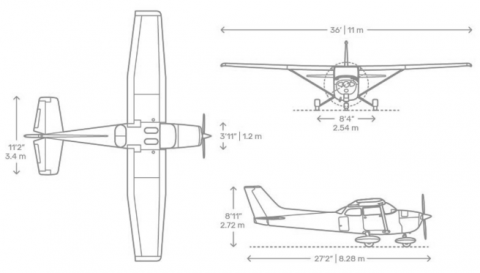Figure 1. Cessna 172 3-view drawing

Table 1. General Aircraft Cessna 172 Skyhawk specifications

 General Aircraft Cessna 172 Skyhawk specifications Wing Span 11 m Horizontal tail span 3.4 m Wing Area 16.2 m2 Horizontal tail area 3.74 m2 Aspect Ratio 7.32 H.T root chord 1.4 m Taper Ratio 0.672 H.T tip chord 0.8 m Wing Root Chord 1.625 m Vertical tail area 1.81 m2 Wing tip chord 1.092 m Fuselage length 8.28 m Sweep Angle 0 o Power plant 1x Lycoming IO-360-L2A, 4 cylinder, max hp (160), (120 kw) Main Aerodynamic chord 1.47m Stall speed with full flaps down 87 km/hr Cross section airfoil NACA 2412 Stall speed without flaps 90 km/hr Zero lift angle -2.07o Airfoil lift slope curve 0.105 deg-1 Wing load 68.6 kg/m2

4.1 Main half wing and proposed modified half wing of an aircraft (Cessna 172)

The main half wing of an aircraft was designed by Solid work (2015) software according to the design data and dimensions of the aircraft as shown in Figure 2. The proposed idea is to create a tangential suction slot (2.6) m length and (30) mm opening width on the upper wing surface at (70%) of the wing chord from the leading edge closed to the wingtip connected with the main suction header tube length (2.6) m and long diameter (120) mm and short diameter (80) mm is built inside the wing as shown in Figures 3 and 4, to absorb and reduce partially adverse static pressure occur in the turbulent flow over the wing at high angle of attacks and delay separation point of the reverse flow to the leading edge of the wing at stall speed without flaps. Depending on the deferential static pressure magnitudes between the upper wing surface caused by adverse pressure and static pressure occurred by flow vortices on the side surface of the wingtip without using absorption equipment.

## 2.png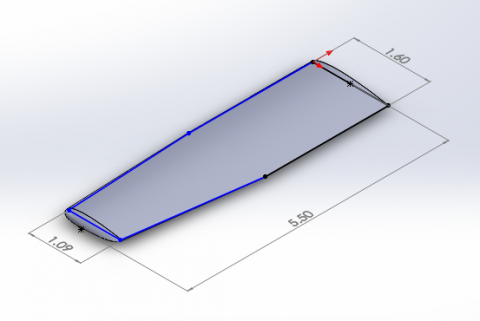Figure 2. Half wing geometry Cessna 172 Skyhawk

## 3.png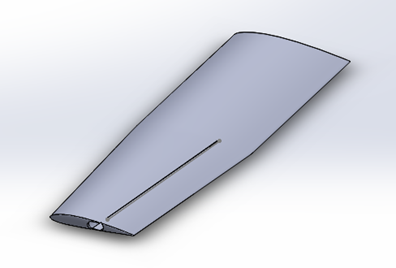Figure 3. Tangential suction slot

## 4.png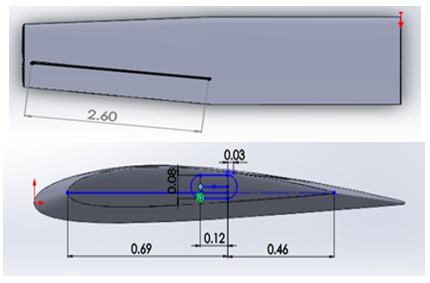Figure 4. Proposed suction tube dimensions

5. Approach Case Study

At high angle of attack, adverse static pressure gradient increased on the upper wing surface, separation point moves to the leading edge and breaks up airflow pattern velocity shape away from the upper surface. This is the natural phenomenon of the airflow viscous responsible increased wing drag force as affected by the skin friction during increasing angle of attack. In this approach consider the tangential suction slot one of the techniques to control backflow airstream flow by partially reduction of adverse static pressure intensity and expelling it through the wing tips ends without using any suction source depending on different static pressure magnitude on these two levels. Computer Fluid Dynamic program (CFD) has been used to investigate and study the case study the effect of tangential suction slot channel to enhance aerodynamic performance of the airplane wing at high angle of attacks with low speed.

6. Governing Equation

Navier-Stokes for unsteady, incompressible, three-dimensional viscous flow is describing the motion of viscous fluid substances where equations are three components of the momentum Equation (1), (2) and (3). However, to close the problem they also contain the continuity equation (4) according to Anderson Jr. . Three components of the momentum equations are:

$\frac{\partial u}{\partial t}+\rho u \frac{\partial u}{\partial x}+\rho v \frac{\partial u}{\partial y}+\rho \omega \frac{\partial u}{\partial z}=-\frac{\partial p}{\partial x}+\frac{\partial}{\partial x}(\lambda \nabla \cdot \vec{V}+$

$\left.2 \mu \frac{\partial u}{\partial x}\right)+\frac{\partial}{\partial y}\left[\mu\left(\frac{\partial v}{\partial x}+\frac{\partial u}{\partial y}\right)\right]+\frac{\partial}{\partial z}\left[\mu\left(\frac{\partial v}{\partial z}+\frac{\partial u}{\partial x}\right)\right]$        (1)

$\rho \frac{\partial v}{\partial t}+\rho u \frac{\partial v}{\partial x}+\rho v \frac{\partial v}{\partial y}+\rho \omega \frac{\partial v}{\partial z}=-\frac{\partial p}{\partial y}+\frac{\partial}{\partial x}\left[\mu\left(\frac{\partial v}{\partial x}+\right.\right.$

$\left.\left.\frac{\partial u}{\partial y}\right)\right]+\frac{\partial}{\partial y}\left(\lambda \nabla \cdot \vec{V}+2 \mu \frac{\partial v}{\partial y}\right)+\frac{\partial}{\partial z}\left[\mu\left(\frac{\partial w}{\partial y}+\frac{\partial v}{\partial z}\right)\right]$         (2)

$\rho \frac{\partial w}{\partial t}+\rho u \frac{\partial w}{\partial x}+\rho v \frac{\partial w}{\partial y}+\rho \omega \frac{\partial w}{\partial z}=-\frac{\partial p}{\partial z}+\frac{\partial}{\partial x}\left[\mu\left(\frac{\partial u}{\partial z}+\right.\right.$

$\left.\left.\frac{\partial w}{\partial x}\right)\right]+\frac{\partial}{\partial y}\left[\mu\left(\frac{\partial w}{\partial y}+\frac{\partial v}{\partial z}\right)\right]+\frac{\partial}{\partial z}\left(\lambda \nabla \cdot \vec{V}+2 \mu \frac{\partial w}{\partial z}\right)$        (3)

The continuity equation is given by:

$\frac{\partial \rho}{\partial t}+\frac{\partial(\rho u)}{\partial x}+\frac{\partial(\rho v)}{\partial y}+\frac{\partial(\rho w)}{\partial z}=0$          (4)

Lift and drag coefficient can be obtained by [6, 9]:

$C_{L}=\frac{1}{C} \int_{0}^{C}\left(C_{p, 1}-C_{p, u}\right) d x$       (5)

$C_{D}=\frac{1}{C} \int_{0}^{C}\left(C_{p, 1} \frac{d y}{d x}-C_{p, u} \frac{d y}{d x}\right) d x$       (6)

$C_{P}=\frac{P-P_{\alpha}}{\frac{1}{2} \rho v^{2}}$        (7)

$R e=\frac{V_{\infty} C \rho_{\infty}}{\mu}$        (8)

7. Numerical Analysis

Computer Fluid Dynamic (CFD) was performed to numerical analysis in this approach to calculate coefficients of lift and drag (CL and CD) respectively, (CL/CD) and (CD/CL) at different high angle of attacks between (10°-24°). Spallart Allmaras' turbulence model was selected; it is a one equation model developed exclusively for applications of aerospace; It solves a kinematic eddy viscosity transport equation . To discretize the equations of the governing, a second-order upwind technique was used, and the SIMPLE method was employed to solve them.

7.1 Boundary condition

Numerical simulation of both half wings was conducted under the same boundary condition. Airflow boundary fluent enclosure has been created by dimension (X=18 m, Y=12 m, and Z=7 m) as shown in Figure 5 to avoid the boundary layers effect on stream flow according to study of Attia et al. .

## 5.png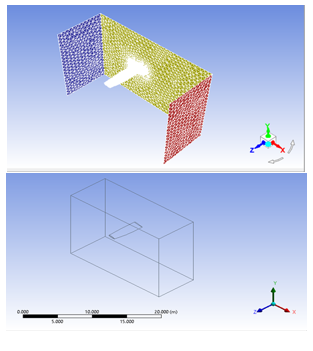Figure 5. Airflow boundary enclosure

where, the boundary condition of the numerical analysis was performed by airstream:

• Inlet Velocity is stall velocity without flaps V = 25 m/s.
• Density of airflow ρ=1.225 kg/m3.
• Temperature  of airflow T=288.15 K.
• Air Pressure at the inlet P=101325 Pa.
• Kinematic viscosity v=0.0001460735 m2/s.
• Reynolds Number Re=2.51 x 105.
• At the outlet boundary, the pressure was fixed to ensure continuity in the pressure system. The outlet air pressure was 101325 Pa.

7.2 Mesh generation and grid independence test

The nodes number and elements number affect the results accurateness and the required time for the simulation. The larger the number of cells, needs more computing time and the longer convergence time required. The main basic half wing model of the Cessna 172 aircraft was meshed by ANSYS workbench software through using tetrahedrons method, inflation, Curvature and Patch independent. On a basic wing model with (0°) angle attack, a grid independent test (GIT) for the mesh was performed to select the appropriate number of elements and nodes in order to obtain highly precise outcomes and reducing simulation time and which would then be applied on the models of the basic wing and a modified wing with different attack angles. Six various meshing cases were opted to evaluate the Grid independent test as shown in Figure 6 . The GIT for the six cases was attained by comparing the results of drag coefficient (CD) on the basic wing model according to the nodes number and elements number as shown in Table 2. The results of six cases are plotted as shown in Figure 7, was observed match results of the drag coefficient (CD) according to the number of elements for the cases (3, 4, 5, and 6). Case (3) was selected to provide precise findings while decreasing simulation period depending on the significant effect on the Central Processing Unit (CPU) of computational time which is Figure 8 as mentioned in Ref. .

Table 2. The elements and nodes number applied in the verification of the GIT

 Case Number Nodes Elements CD Case (1) 228708 823143 0.00288 Case (2) 414690 1481840 0.01221 Case (3) 516077 1949924 0.01193 Case (4) 618075 2266161 0.01210 Case (5) 701033 2655588 0.01191 Case (6) 802274 3129037 0.01190

## 6.png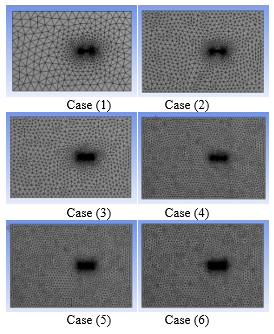Figure 6. Meshing cases for conducting the GIT

## 7.png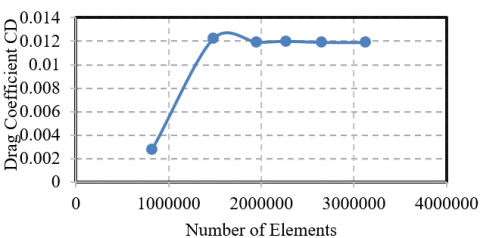Figure 7. Grid independence test results for drag coefficient main half wing

## 8.png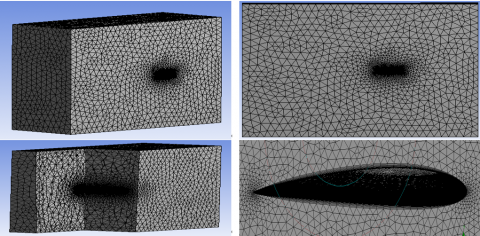Figure 8. Case (3) Meshing detail of the model

8. Results and Discussions

Numerical Analysis was been performed on the basic half wing and the proposed modification of the aircraft. The results of the analytical simulation are shown in Table 3 which are represent drag coefficient (CD), lift coefficient (CL), wing efficiency as a ratio of lift to drag coefficients, these parameters are affected by increasing angle of attacks. The effect of the suction slot on the lift and drag coefficients at high angle of attacks between (10° and 24°) as a selected angle of attacks at the stall velocity without using flaps is (25 m/s). The suction slot affected to increase aerodynamic efficiency of the wing by partially sucking adverse backflow pressure and delayed forward movement of separation point than the original main wing. Figures 9-12 are graphs of represents relationship between the CL, CD as a lift and drag coefficients respectively, and CL/CD and CD/CL are ratio lift-to-drag coefficients as a wing efficiency at a selected angle of attacks respectively, Figure 13 is plot of relationship between CL and CD which is represent the drag polar of the wing.

## 9.png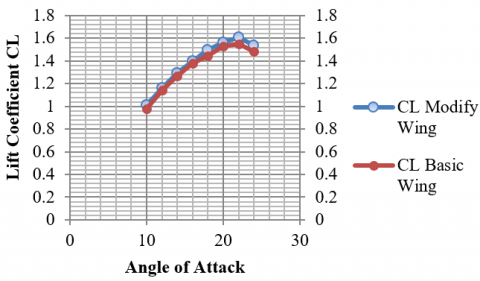Figure 9. Lift Coefficient CL Vis AoA

## 10.png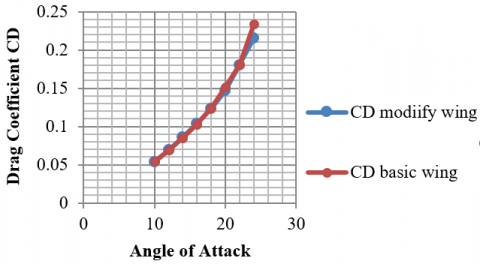Figure 10. Drag Coefficient CD Vis AoA

## 11.png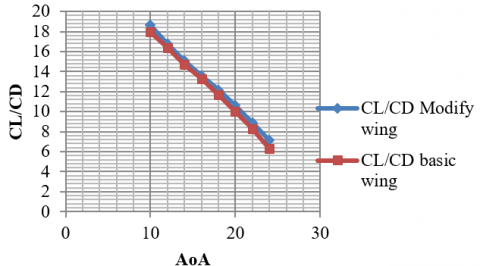Figure 11. Wing Efficiency CL/CD Vis AoA

## 12.png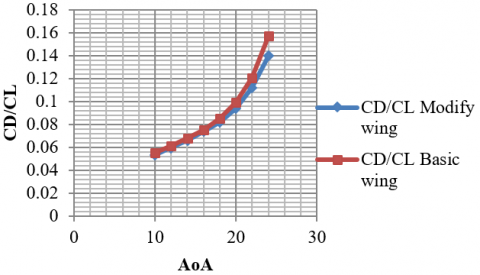Figure 12. Wing Efficiency CD/CL Vis AoA

## 13.png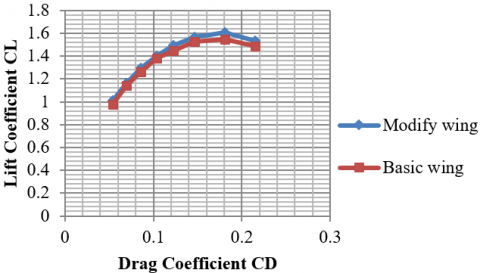Figure 13. Drag Polar CL Vis CD

As demonstrated in Figures 9-13 and Tables 3-6, when the angle of attack is increased, lift Coefficient (CL), drag coefficient (CD), and wing efficiency (CL/CD) increase, while the (CD/CL) reduces. Moreover, the percentage rates ratio of change values of the CL between (10 to 24) degrees of angle of attacks are (3.757%) and the percentage rates ratio of change values of the CD between (10 to 24) degrees of angle of attacks is (-0.530%).

Table 3. Drag coefficient, lift coefficient and the ratio of lift to drag for the both wing models

 AoA (Degree) Basic Half wing Modified Half wing with slot CL CD CL /CD CL CD CL / CD 10 0.97795 0.05439 17.98032727 1.0092 0.05413 17.98032727 12 1.14058 0.06964 16.3782309 1.16163 0.06933 16.52056779 14 1.26501 0.08596 14.71626338 1.29312 0.08562 14.81970478 16 1.37827 0.10396 13.25769527 1.40112 0.10296 13.38646076 18 1.44739 0.12387 11.68475014 1.49508 0.12275 11.68475014 20 1.52708 0.15196 10.04922348 1.56536 0.14693 10.04922348 22 1.54828 0.18662 8.296431251 1.60912 0.18054 8.296431251 24 1.48611 0.234 6.090614754 1.53659 0.21527 6.350897436

Table 4. Represent a change of values CL, CD, CL/CD and CD/CL between the two models indicated as ΔCL. ΔCD, Δ(CL/CD) and Δ(CD/CL) depending on a selected angle of attacks

 AoA (Degree) ΔCL ΔCD Δ(CL/CD) Δ(CD/CL) 10 1.031955 0.99522 1.036911 -0.9644 12 1.018456 0.995549 1.02301 -0.97751 14 1.022221 0.996045 1.02628 -0.97439 16 1.016579 0.99471 1.02198 -0.97849 18 1.032949 0.990958 1.04237 -0.95935 20 1.025067 0.966899 1.06016 -0.94325 22 1.039295 0.96742 1.07429 -0.93084 24 1.033968 0.882254 1.17196 -0.85327

Table 5. Represents the percentage change in parameter values due the effect tangential suction slot for a selected angle of attacks

 AoA (Degree) ΔCL% ΔCD% Δ(CL/CD)% Δ(CD/CL)% 10 3.125 -0.026 3.691 -3.556 12 2.105 -0.031 2.301 -2.249 14 2.811 -0.034 2.628 -2.561 16 2.285 -0.055 2.198 -2.151 18 4.769 -0.112 4.237 -4.065 20 3.828 -0.503 6.016 -5.675 22 6.084 -0.608 7.429 -6.916 24 5.048 -2.873 17.196 -14.673

Table 6. Represent the percentage ratio of change parameter values

 ΔCL % ΔCD % Δ(CL/CD)% Δ(CD/CL)% 3.757 -0.530 5.712 -5.231

## 14.png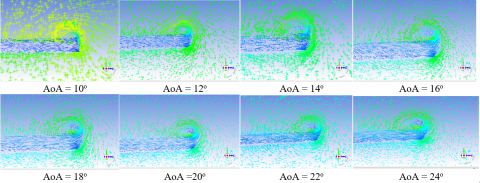Figure 14. Absorption effect of the tangential sucking slot on adverse pressure

AoA

Main half wing Pressure Contour

Modified half wing Pressure Contour

Scale

10

## 15.1.png## 15.2.png## 15.17.png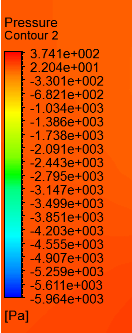12

## 15.3.png## 15.4.png14

## 15.5.png## 15.6.png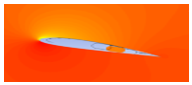16

## 15.7.png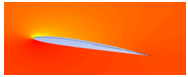## 15.8.png18

## 15.9.png## 15.10.png20

## 15.11.png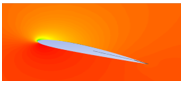## 15.12.png22

## 15.13.png## 15.14.png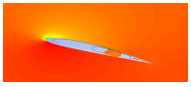24

## 15.15.png## 15.16.png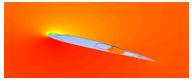Figure 15. Pressure Contour

The difference in static pressure arising between the upper surface of the wing at high angle of attacks because of the adverse pressure and the tip of the wing led to absorption partially backflow of the air stream from the upper surface through the suction header tube by the tangential suction slot to discharge it through the ends of the wing, as a resultant that reduction partially adverse pressure and restricted forward movement of the separation to the leading edge as shown in Figure 15. The suction flow is shown in Figure 14 due to the cross-section plan created by the CFD in section the suction header tube for a chosen angle of attack between (10° to 24°).

9. Conclusions

In this research, the case study was implemented on a half wing geometry of aircraft Cessna 172. To improve wing efficiency at limited flight condition with critical stall speed (90 km/hr.) and stall angle of attack (24°) without using lift devices (flaps) according to the aircraft data, to maintain a steady level flight. Numerical analysis was performed using (CFD) software to analyze influence tangential suction slot on the upper surface at 70% of the chord measuring from the leading edge to energized the kinetic energy to control the airflow on the upper surface of the wing in a turbulent boundary layer by absorbing part of the adverse pressure generated at high angle of attacks physically without absorbing machines or equipment depending on the deferential static pressure magnitudes between the upper wing surface caused by adverse pressure and static pressure occurred by flow vortices on the side surface of the wingtip The effect of this technique on the lift coefficient CL and drag coefficient CD magnitudes as a main parameter to determine the wing efficiency as a lift-to drag ratio and wing drag polar as a relationship between lift coefficient to the drag coefficient to improve the wing performance at high angle of attacks between (10° to 24°) degree where the angle of attack (24°) is a stall angle of attack. The results show the percentage rates ratio of change values in lift coefficient CL is increased to (3.75%) and drag coefficient CD is reduced to (-0.530%) at a chosen angle of attacks between (10° to 24°) degrees, the wing efficiency ratio related to the Δ(CL/CD) is increased to (5.712%). Stall speed is reduced by 2.22% to be (88 km/hr.). The results of this research case study are improved aircraft Cessna 172 performance at critical angle of attack with stall velocity to maintain the aircraft at the straight steady flight and maneuvers with high angle of attacks and reduce landing distance.

Acknowledgment

The authors would like to thank the University of Baghdad, Iraq, for their assistance and support.

References

 Yousefi, K., Saleh, R., Zahedi, P. (2014). Numerical study of blowing and suction slot geometry optimization on NACA 0012 airfoil. Journal of Mechanical Science and Technology, 28(4): 1297-1310. https://doi.org/10.1007/s12206-014-0119-1

 Greenblatt, D., Wygnanski, I.J., Rumsey, C.L. (2010). Aerodynamic flow control. Encyclopedia of Aerospace Engineering. 1: 3-12. https://doi.org/10.1002/9780470686652.eae019

 Bashir, M., Rajendran, P., Sharma, C., Smrutiranjan, D. (2018). Investigation of smart material actuators & aerodynamic optimization of morphing wing. Materials Today: Proceedings, 5(10): 21069-21075. https://doi.org/10.1016/j.matpr.2018.06.501

 Mousa, N.A.R. (2014). Proposed modification to increase main swept back wing efficiency for aircraft Aermacchi Siai S211. Journal of Engineering, 20(10). https://www.iasj.net/iasj/article/93552.

 Ali, A.H. (2017). Aerodynamic characteristics of a rectangular wing using non-linearvortex ring method. Journal of Engineering, 23(4): 125-141. https://joe.uobaghdad.edu.iq/index.php/main/article/view/55.

 Schlichting, H. (1968). Boundary Layer Theory McGraw Hill Book Co. New York.

 Gad-el-Hak, M. (1996). Modern developments in flow control. Applied Mechanics Reviews, 49(7): 365-379. https://doi.org/10.1115/1.3101931

 Huang, L., Huang, P.G., LeBeau, R.P., Hauser, T. (2004). Numerical study of blowing and suction control mechanism on NACA0012 airfoil. Journal of Aircraft, 41(5): 1005-1013. https://doi.org/10.2514/1.2255

 Shojaefard, M.H., Noorpoor, A.R., Avanesians, A., Ghaffarpour, M. (2005). Numerical investigation of flow control by suction and injection on a subsonic airfoil. American Journal of Applied Sciences, 2(10): 1474-1480. https://doi.org/10.3844/ajassp.2005.1474.1480

 Beliganur, N.K., Raymond, P. (2007). Application of evolutionary algorithms to flow control optimization. Report of University of Kentuchky. https://uknowledge.uky.edu/cgi/viewcontent.cgi?article=1454&context=gradschool_theses.

 Jasim, L.M. (2008). A numerical study of the unsteady flow separation over an airfoil with and without suction and blowing as an active boundary layer control (Doctoral dissertation, Ph. D. Thesis, Mosul University, College of Engineering, Mech. Dep).

 Jensch, C., Pfingsten, K.C., Radespiel, R. (2010). Numerical investigation of leading edge blowing and optimization of the slot geometry for a circulation control airfoil. In New Results in Numerical and Experimental Fluid Mechanics VII, pp. 183-190. https://doi.org/10.1007/978-3-642-14243-7_23

 Genç, M.S., Kaynak, Ü., Yapici, H. (2011). Performance of transition model for predicting low Re aerofoil flows without/with single and simultaneous blowing and suction. European Journal of Mechanics-B/Fluids, 30(2): 218-235. https://doi.org/10.1016/j.euromechflu.2010.11.001

 Piperas, A.T. (2010). Investigation of boundary layer suction on a wind turbine airfoil using CFD. Technical University of Denmark.

 Ravindran, S.S. (2000). Active control of flow separation over an airfoil. Langley Research Center, Hampton, Virginia. https://www.academia.edu/3534912/Active_Control_of_Flow_Separation_Over_an_Airfoil.

 Petrov, A.G., Sukhov, A.D. (2021). Laminar flow of viscous fluid around elliptical contours under angle of attack. arXiv preprint arXiv:2106.14017. https://doi.org/10.48550/arXiv.2106.14017

 McNeal, G.S. (2016). Drones and the future of aerial surveillance. Geo. Wash. L. Rev., 84: 354.

 Vadivelu, P., Lakshmanan, D., Naveen, R., Mathivannan, K., Kumar, G.Y. (2020). Numerical study on Longitudinal control of Cessna 172 Skyhawk aircraft by Tail arm length. In IOP Conference Series: Materials Science and Engineering, 764: 012026. https://doi.org/10.1088/1757-899X/764/1/012026

 Anderson Jr, J.D. (2010). Fundamentals of Aerodynamics. Tata McGraw-Hill Education.

 Kostić, Č. (2015). Review of the Spalart-Allmaras turbulence model and its modifications to three-dimensional supersonic configurations. Scientific Technical Review, 65(1): 43-49. https://doi.org/10.5937/STR1501043K

 Attia, O.H., Kazum, A.A., Adam, N.M. (2021). Numerical investigation for suitable small-scale wind turbine blade aerodynamically for remote areas. Journal of Mechanical Engineering Research and Developments, 44(4): 381-391. https://jmerd.net/04-2021-381-391

 Mahmood, H.A., Al-Sulttani, A.O., Attia, O.H., Adam, N.M. (2021). A numerical study to improve the position and angle of the producer gas injector inside the intake manifold to minimize emissions and efficiency enhancement of a bi engine. EUREKA: Physics and Engineering, (5): 100-109.‏ https://doi.org/10.21303/2461-4262.2021.002045.

 Mahmood, H.A., Al-Sulttani, A.O., Attia, O.H. (2021). Simulation of syngas addition effect on emissions characteristics, combustion, and performance of the diesel engine working under dual fuel mode and lambda value of 1.6. The IOP Conference Series: Earth and Environmental Science, 779: 012116. https://doi.org/10.1088/1755-1315/779/1/012116